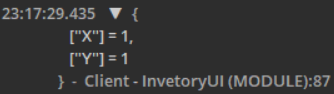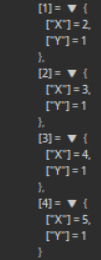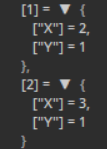# Grid-based Inventory; I've made a matrix, now what?

In order to create a grid-based inventory (e.g, this example), I’ve created a matrix based on the number of grids in the player’s backpack. E.g:

``````local InventoryTable = {
{0, 0, 0, 0},
{0, 0, 0, 0},
{0, 0, 0, 0},
{0, 0, 0, 0},
}
``````

Where zero represents an open grid (and 1 being occupied).
However, when the player does pick up an item, how would I recognize and position the item UI over an available part of the grid?

4 Likes

So if I’m understanding you correctly, this grid is supposed to represent 16 different cubes where stuff goes in you inventory. You want to know how to find the positions of this grid on the screen. If you look at the person example in the video, they have grey squares for their grid. You should do this as well, make a separate GUI for every 0 and reference it’s position. For example you could make an invisible GUI on the upper left corner of the gui, and use it as the first 0’s position. So when someone picks something up, if the first 0 in the table is 0, it will put a picture of the thing they picked up (I’m assuming you can do that) where the corresponding GUI is and turn the 0 to 1. Now if you’re talking about how they did the multiple square items, you can just take two squares, and turn two 0s to ones (putting the image of the item in between the two). This might be unprofessional to say, and I know that big block of writing might be confusing, so I will provide examples later when I can respond thoroughly.

1 Like

You can represent the space that each item takes up as a similar grid:

``````local stoneInvShape = {
{ 1 }
}
local daggerInvShape = {
{ 1 },
{ 1 },
}
local scytheInvShape = {
{ 1, 1, 1 },
{ 0, 0, 1 },
{ 0, 0, 1 },
{ 0, 0, 1 },
}
``````

You can then test if a shape fits into a grid like this:

``````
local SLOT_EMPTY = 0
local SLOT_FILLED = 1

function shapeFitsInGridAtPoint(shape, grid, gridPointX, gridPointY)
--Check that every 1 in the shape fits inside the grid and doesn't overlap with 1s, if the shape were to be placed with its upper left point at (gridPointX, gridPointY).
for shapeX = 1, #shape do
for shapeY = 1, #shape do
local gridX, gridY = gridPointX + shapeX, gridPointY + shapeY

--If a point in the shape is outside the grid, then it doesn't fit
if gridX < 1 or gridY < 1 or gridX > #grid or gridY > #grid then
return false
end

--0s in the grid have no effect on whether the shape fits or not
if grid[gridY][gridX] == SLOT_EMPTY then continue end

--Neither do 0s in the shape
if shape[y][x] == SLOT_EMPTY then continue end

--If both the shape and the grid have 1s at this point, there's an overlap so shape doesn't fit here
return false
end
end

--None of the 1s in the shape overlapped with 1s in the grid, so the shape fits
return true
end

function getPointWhereShapeFitsInGrid(shape, grid)
for gridX = 1, #shape do
for gridY = 1, #shape do
if shapeFitsInGridAtPoint(shape, grid, gridX, gridY) then
return {X = gridX, Y = gridY}
end
end
end

return nil
end
``````

y and x here is not defined in the rest of the script, am I right in assuming y and x here is just shapeX and shapeY?

Also, is this line only necessary for tool shapes such as the example below?

1 Like

Also what exactly should be returned when going through the functions?
This is the shape of an example tool I’m using.

``````	self.ToolShape = {
{1},
{1},
{1},
{1},
}
``````

And this is the result I get printed out.Not exactly sure what I’m seeing here.

1 Like

Bumping this post again, any response would be good.

1 Like

To the best of my knowledge - considering the context which is given - yes, the x and y variables refer to shapeX and shapeY, respectively.

What type of tool shapes are you planning to use? This is intended to be - I believe - used for any tool that can be described using a matrix of zeros and ones. It can support any dimensions of shapes, whether if it’s a column or row of 1s, or if something more complex. This way, you can add any new items in the case of, say, an update. If this is not what you want, you can optimize @ThanksRoBama’s solution to your specific shapes of inventories.

The return value is essentially the first available point in which the shape can be fit into the grid. That is, we can place the shape (starting from the top left corner) into the returned coordinates and it will not cause any problems with fitting.

If you want a list of points where you can place your shape, for example, you can append any matching points into an array and return that array containing your results.

``````function getPointWhereShapeFitsInGrid(shape, grid)
local points = {}
for gridX = 1, #shape do
for gridY = 1, #shape do
if shapeFitsInGridAtPoint(shape, grid, gridX, gridY) then
table.insert(points, {X = gridX, Y = gridY})
end
end
end

return points
end

-- Unittesting
local urShape = ur.Shape
local urGrid = ur.Grid
for _, coordinate in ipairs(getPointWhereShapeFitsInGrid(urShape, urGrid)) do
print(coordinate.X, ",", coordinate.Y)
end
``````
1 Like
``````local InvenTable =
{
{1, 0, 0, 0},
{0, 0, 0, 0},
{0, 0, 0, 0},
{0, 0, 0, 0},
}

self.ToolShape = {
{1},
{1},
{1},
{1},
}
``````

I tried testing the code out with the first top left spot being already occupied.
This returns no results. How would I go about checking the next spot available going from left to right?

1 Like

Can I see your implementation? I haven’t tested the code that was posted, so I’ll try doing some testing and get back to you.

1 Like
``````local InvenTable =
{
{1, 0, 0, 0, 0},
{0, 0, 0, 0, 0},
{0, 0, 0, 0, 0},
{0, 0, 0, 0, 0},
}

local InventoryUI = {}

InventoryUI.__index = InventoryUI

function InventoryUI.new(tool)
local self = setmetatable({}, InventoryUI)

self.Tool = tool
self.ToolShape = {
{1},
{1},
{1},
{1},
}
return self
end

function InventoryUI:CreateFrame()	-- Create frame for the item
self:FindAvailableSpace()
end

function InventoryUI:ItemFitsIndividualPoint(gridPointX, gridPointY)
for ToolShapeX = 1, #self.ToolShape do
for ToolShapeY = 1, #self.ToolShape do
local gridX, gridY = gridPointX + ToolShapeX, gridPointY + ToolShapeY

--If a point in the shape is outside the grid, then it doesn't fit
if gridX < 1 or gridY< 1 or gridX > #InvenTable or gridY > #InvenTable then
return false
end

--0s in the grid have no effect on whether the shape fits or not
if InvenTable[gridY][gridX] == 0 then continue end

--Neither do 0s in the shape
if self.ToolShape[ToolShapeY][ToolShapeX] == 0 then continue end

--If both the shape and the grid have 1s at this point, there's an overlap so shape doesn't fit here
return false
end
end

--None of the 1s in the shape overlapped with 1s in the grid, so the shape fits
return true
end

function InventoryUI:FindAvailableSpace()
for gridX = 1, #self.ToolShape do	-- gridX is the first row
for gridY = 1, #self.ToolShape do	-- Columns across the first row going down
if self:ItemFitsIndividualPoint(gridX, gridY) then
print(gridX, gridY)
end
end
end
end

return InventoryUI
``````
1 Like

I modified the original implementation, accounting for edge cases. Try the following:

``````local SLOT_EMPTY = 0
local SLOT_FILLED = 1

function shapeFitsInGridAtPoint(shape, grid, gridPointX, gridPointY)
--Check that every 1 in the shape fits inside the grid and doesn't overlap with 1s, if the shape were to be placed with its upper left point at (gridPointX, gridPointY).
for shapeX = 1, #shape do
for shapeY = 1, #shape do
local gridX, gridY = gridPointX + shapeX - 1, gridPointY + shapeY - 1

--0s in the grid have no effect on whether the shape fits or not
if grid[gridY][gridX] == SLOT_EMPTY then continue end

--Neither do 0s in the shape
if shape[shapeY][shapeX] == SLOT_EMPTY then continue end

--If both the shape and the grid have 1s at this point, there's an overlap so shape doesn't fit here
return false
end
end

--None of the 1s in the shape overlapped with 1s in the grid, so the shape fits
return true
end

function getPointWhereShapeFitsInGrid(shape, grid)
local points = {}
for gridX = 1, #grid-#shape+1 do
for gridY = 1, #grid-#shape+1 do
if shapeFitsInGridAtPoint(shape, grid, gridX, gridY) then
table.insert(points, {X = gridX, Y = gridY})
end
end
end

return points
end
``````
3 LikesThis is the result printed out from the points table.
I just wanted to confirm the keys in the points table are the individual points from the tool shape and the values are the co-ords from the inventory matrix?

Also:

I meant tool shapes as in shapes like a scythe in this example:

Would that require a rectangular shape with 0’s in the tool shape matrix, or can it be written as:

``````local scytheInvShape = {
{ 1, 1, 1 },
{ 1 },
{ 1 },
{ 1 },
}
``````

I’m assuming not, but I’m afraid of situations where there’s already an item in the player’s inventory, and to fit the scythe into the player inventory, the code wouldn’t work due to the 0’s in the item’s shape.

1 Like

The index simply is an address that points to a coordinate in which the shape can fit into the grid - it is just an arbitrary number. The value, as you say, is the respective coordinate from the grid matrix (keep in mind that the literal starting point that the coordinate is referring would be `grid[coordinate.Y][coordinate.X]`.

The shape does need to be rectangular, which is why we include zeros in the areas where the shape does NOT take up space. In other words, it would, in a graphical sense, be identical to:

``````local scytheInvShape = {
{ 1, 1, 1 },
{ 1 },
{ 1 },
{ 1 },
}
``````

If you really wanted to use a shape like the above, you can simply check if the element that is indexed in the shape is `nil` (in the `shapeFitsInGridAtPoint` function), and then break out of the inner loop. In my opinion, this type of structure is superfluous, and it is better include the zeros for the sake of clarity and simplicity.

1 Like

However, when running the code again with the same inventory table and a tool shape of:

``````	self.ToolShape = {
{1, 1, 1},
{1, 0, 0},
{1, 0, 0},
{1, 0, 0},
}
``````

The resulting points table prints out only two indexes?1 Like

I think this is expected? Based on the code you have given previously, (2, 1) and (3, 1) seem to be the only valid coordinates. I am not sure how the previous result outputted more coordinates. Can you run more tests and get back to me?

1 Like

The previous result of

``````local scytheInvShape = {
{ 1, 1, 1 },
{ 1 },
{ 1 },
{ 1 },
}
``````

did not print out any more results, in fact, it was the same results as the ones including the 0’s in the tool shape.
But this only gives coordinates to two points in the tool shape, how is this to be expected? Shouldn’t it print out co-ords for all of the points in the tool shape?

Also adding onto this, I tested more item shapes that contained more than one value horizontally.

``````	self.ToolShape = {
{1, 1},
}
``````

The code appears to not work in this scenario.

1 Like

The coordinates is not related to the contents of the shape, but rather the point (which would be the top left corner of the shape) where the shape can legally fill the grid.

Note that the results here would be displayed in the output as {X = 1, Y = 1} and {X = 2, Y = 1}, respectively.

1. In that case, how would I change the values of the inventory matrix so that the shape is placed in it?
2. I still don’t see why
``````	self.ToolShape = {
{1, 1},
}
``````

would print out this result, could you explain?

1 Like

You would iterate starting from the coordinate that you obtained to the bottom right of the shape, iterating through the elements (0s and 1s) of the shape.

``````local coord = {X = something, Y = somethingElse}
for i = 1, #shape do
for j = 1, #shape[i] do
grid[coord.Y+i-1][coord.X+j-1] = shape[i][j]
end
end
``````

Admittedly, this will take a bit to write out as a diagram. Essentially, picture your 4x5 inventory table, and using the coordinate system in the diagram I’ve shown above, circle all the 1x2 group of 0s (all the groups that can be filled with your item) in that diagram. Write down every top left coordinate of each group, what you should end up with is an array of coordinates whose elements are equal to the elements shown in your output.

1 Like

This works great, however, for item shapes such as

``````	self.ToolShape = {
{1, 1},
}
``````

How would I prevent it from filling up the entire inventory matrix?

Edit: Scratch that, I’ve fixed it. If anyone wants to know the solution just ask as it needs a bit of explaining.

1 Like